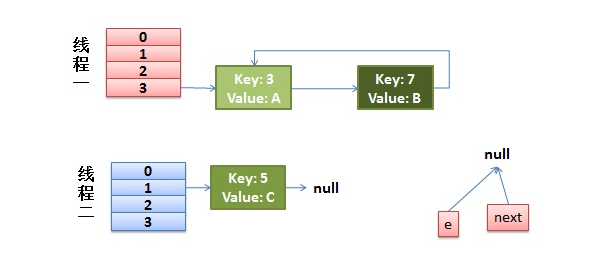# 1、HashCode

## 1.1、一些常见的 HashCode

### 1.1.1、Integer


@Test
public void Integer_HashCode(){

Integer one  = new Integer(20);
System.out.println(one.hashCode()); //20
}

/**
* Integer 的 hashCode 就是它的value
*
*     public int hashCode() {
*         return Integer.hashCode(value);
*     }
*/


### 1.1.2、String


@Test
public void String_HashCode(){
String str1  ="123";
System.out.println(str1.hashCode()); // 48690

}

/**
* ASCII http://tool.oschina.net/commons?type=4

* String 类的散列值就是依次遍历其每个字符成员，
* 递归的将当前得到的hashCode乘以31然后加上下一个字符成员的ASCII值 （h = 31 * h + val[i];）
*
*   h 初始为 0
*  '1'  49   h = 31 * 0  + 49 = 49
*  '2'  50   h = 31 * 49 + 50 = 1569
*  '3'  51   h = 31 * 1569 + 51 = 48690
*
*     public int hashCode() {
*         int h = hash;
*         if (h == 0 && value.length > 0) {
*             char val[] = value;
*
*             for (int i = 0; i < value.length; i++) {
*                 h = 31 * h + val[i];
*             }
*             hash = h;
*         }
*         return h;
*     }
*/



## 1.2、为什么HashCode 会使用31

Why does Java’s hashCode() in String use 31 as a multiplier?

The value 31 was chosen because it is an odd prime. If it were even and the multiplication overflowed, information would be lost, as multiplication by 2 is equivalent to shifting. The advantage of using a prime is less clear, but it is traditional. A nice property of 31 is that the multiplication can be replaced by a shift and a subtraction for better performance: 31 * i == (i << 5) - i. Modern VMs do this sort of optimization automatically.

（h = 31 * h + val[i];）



As Goodrich and Tamassia point out, If you take over 50,000 English words (formed as the union of the word lists provided in two variants of Unix), using the constants 31, 33, 37, 39, and 41 will produce less than 7 collisions in each case. Knowing this, it should come as no surprise that many Java implementations choose one of these constants.



#### 2.1、原因，后面可视化也有更详细的解释

1、31是一个不大不小的质数（素数）

2、31可以被 JVM 优化，31 * i = (i << 5) - i。现代的 Java 虚拟机可以自动的完成这个优化

假设 n=3
i=0 -> h = 31 * 0 + val
i=1 -> h = 31 * (31 * 0 + val) + val
i=2 -> h = 31 * (31 * (31 * 0 + val) + val) + val
h = 31*31*31*0 + 31*31*val + 31*val + val
h = 31^(n-1)*val + 31^(n-2)*val + val



——-仅计算公式中次数最高的那一项———–

#### 2.2、可视化得出结论

public static Integer hashCode(String str, Integer multiplier) {
int hash = 0;
for (int i = 0; i < str.length(); i++) {
hash = multiplier * hash + str.charAt(i);
}

return hash;
}

/**
* 计算 hash code 冲突率，顺便分析一下 hash code 最大值和最小值，并输出
* @param multiplier
* @param hashs
*/
public static void calculateConflictRate(Integer multiplier, List<Integer> hashs) {
Comparator<Integer> cp = (x, y) -> x > y ? 1 : (x < y ? -1 : 0);
int maxHash = hashs.stream().max(cp).get();
int minHash = hashs.stream().min(cp).get();

// 计算冲突数及冲突率
int uniqueHashNum = (int) hashs.stream().distinct().count();
int conflictNum = hashs.size() - uniqueHashNum;
double conflictRate = (conflictNum * 1.0) / hashs.size();

System.out.println(String.format("multiplier=%4d, minHash=%11d, maxHash=%10d, conflictNum=%6d, conflictRate=%.4f%%",
multiplier, minHash, maxHash, conflictNum, conflictRate * 100));
}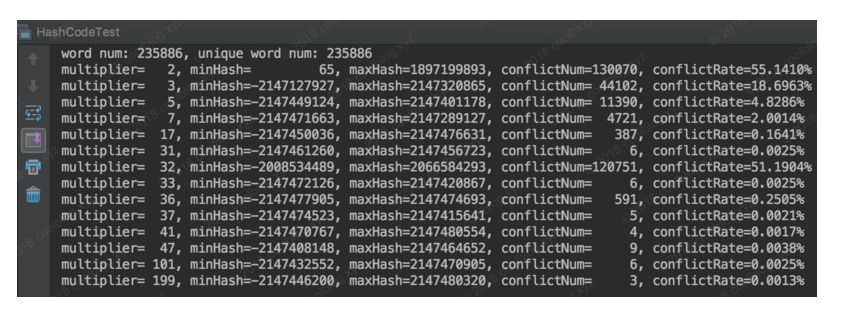1、 质数2、冲突率达到了 55%以上，而且hash值分布不是很广泛，，仅仅分布在了整个哈希空间的正半轴部分，即 0 ~ 2^31-1。而负半轴 -2^31 ~ -1，则无分布。这也证明了我们上面断言，即质数2作为乘子时，对于短字符串，生成的哈希值分布性不佳。

2、奇质数，101、109 表现也不错，冲突率很低，说明了哈希值溢出不一定导致冲突率比较高，但是溢出的话，我们不认为是我们的优选乘子 ,如果不在意质数101容易导致数据信息丢失问题，或许其是一个更好的选择

3、偶数 32、36 这两个并不好，32的冲突率已经超过了50%，尽管36表现好一些，但是和31,37相比，冲突率还是比较高的，但是**偶数也不一定作为乘子冲突率就比较高 **

4、奇质数 31、37 、41 表现都不出，冲突数都小于7个，使用较小的质数做为乘子时，冲突率会很高。 选择比较大的质数作为乘子时，冲突率会降低，但是可能会再次哈希值溢出

## 1.3、HashCode 使用

### 1.3.1、HashCode 特性（确定位置，但不能确定地址）

Object角度看，JVMnew一个Object，它都会将这个Object丢到一个Hash表中去，这样的话，下次做Object的比较或者取这个对象的时候（读取过程），它会根据对象的HashCode再从Hash表中取这个对象。这样做的目的是提高取对象的效率。若HashCode相同再去调用equal。

（1）HashCode 的存在主要是用于查找的快捷性，如HashtableHashMap等，HashCode是用来在散列存储结构中确定对象的存储地址的；

（2）如果两个对象相同， equals方法一定返回true，这两个对象的HashCode一定相同；除非重写了HashCode方法，及其特殊情况下，才回这样重写

（3）两个对象的HashCode相同，并不一定表示两个对象就相同，也就是equals方法不一定返回true，只能够说明这两个对象在散列存储结构中，如Hashtable，他们存放在同一个篮子里。

（4）如果对象的equals方法被重写，那么对象的HashCode也尽量重写，并且产生HashCode使用的对象，一定要和equals方法中使用的一致，否则就会违反上面提到的第2点；

### 1.3.2、HashCode 作用

Java中的集合（Collection）有两类，一类是List，再有一类是Set。前者集合内的元素是有序的，元素可以重复；后者元素无序，但元素不可重复。

equals方法可用于保证元素不重复，但是，如果每增加一个元素就检查一次，如果集合中现在已经有1000个元素，那么第1001个元素加入集合时，就要调用1000次equals方法。这显然会大大降低效率。

（1）如果这个位置上没有元素，它就可以直接存储在这个位置上，不用再进行任何比较了；

（2）如果这个位置上已经有元素了，就调用它的equals方法与新元素进行比较，相同的话就不存了；

（3）不相同的话，也就是发生了Hash key相同导致冲突的情况，那么就在这个Hash key的地方产生一个链表，将所有产生相同HashCode的对象放到这个单链表上去，串在一起（很少出现）。这样一来实际调用equals方法的次数就大大降低了，几乎只需要一两次。 （下面1、的实例就为这里的测试实例）

### 1.3.4、Set测试(equals比较是否相等)

#### 1.3.4.1、实例重写HashCode方法


public class HashTest {
private int i;

public int getI() {
return i;
}

public void setI(int i) {
this.i = i;
}

@Override
public int hashCode() {
return i % 10;
}

/**
*  对象的内存地址与hashcode有关系，但并不是hashcode值相等，就是代表内存地址相同，这种想法是幼稚的
*  比如hashtable中hashcode值相等，
*  	但是存了很多的对象，这表明对象的== 肯定不相等，Ojbect逆向推理，equals不相等，==肯定不相等
*
*/

public final static void main(String[] args) {
HashTest a = new HashTest();
HashTest b = new HashTest();

System.out.println(a.hashCode() == b.hashCode());  //true 人为制造hashcode值相同
System.out.println(a==b);    //false //== 比较对象的相等比较对象引用地址是否相等。还要要比较对象内容是否相等
System.out.println(a.equals(b)); //false 不同的对象 object中 == 和euqals是一样的

a.setI(1);
b.setI(1);
Set<HashTest> set = new HashSet<HashTest>();
//没有 equels 重写的情况
System.out.println(a.hashCode() == b.hashCode());  //true hashcode相同

System.out.println(a.equals(b));    //false 不同的对象 ，创建出来的是地址就不同了

System.out.println(set.size());   //2 这个时候会发想存入了两个值
//放是根据hashcode值存放，如果hashcode值相同，
//再比较equals值，如果equals值也相同，则产生一个单链表放进去

}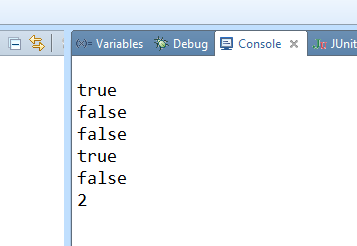#### 1.3.4.2、重写equels方法


public class HashTest {
private int i;

public int getI() {
return i;
}

public void setI(int i) {
this.i = i;
}

// public boolean equals(Object obj) {
//    return this == obj;
// }
@Override
public boolean equals(Object object) {
if (object == null) {
return false;
}
if (object == this) {
return true;
}
if (!(object instanceof HashTest)) {
return false;
}
HashTest other = (HashTest) object;
if (other.getI() == this.getI()) {
return true;
}
return false;
}

@Override
public int hashCode() {
return i % 10;
}
public final static void main(String[] args) {
HashTest a = new HashTest();
HashTest b = new HashTest();

System.out.println(a.hashCode() == b.hashCode());   //true
System.out.println(a==b);       //false 地址不相同
System.out.println(a.equals(b));    //true 重写了equals方法 ，让其相等了

a.setI(1);
b.setI(1);
Set<HashTest> set = new HashSet<HashTest>();

System.out.println(a.hashCode() == b.hashCode());       //true
System.out.println(a.equals(b));    //true   (因为是 || 或者关系也就是说 == 或者 equals 只要成立一个即可)

System.out.println(set.size());     //1

}
}
}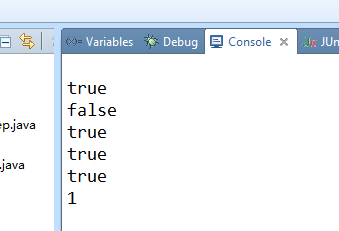# 2、JDK1.8、HashMap

https://blog.csdn.net/v123411739/article/details/78996181

## 2.1、类结构

### 2.1.1、HashMap继承关系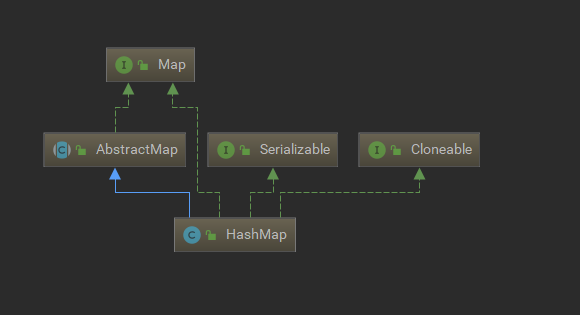public class HashMap<K,V> extends AbstractMap<K,V>
implements Map<K,V>, Cloneable, Serializable {
}


### 2.1.2、HashMap 内部类

#### 2.1.2.1、Node<K,V>：链表节点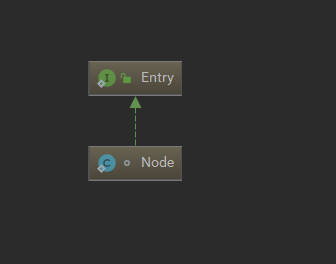// 链表节点, 继承自Entry
static class Node<K,V> implements Map.Entry<K,V> {
final int hash;
final K key;
V value;
Node<K,V> next;

// ... ...
}



#### 2.1.2.2、TreeNode<K,V>：红黑树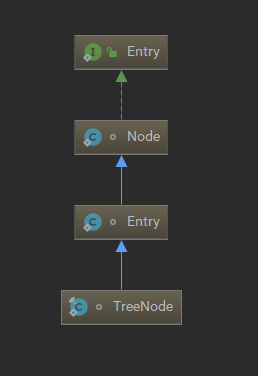内部类

// 红黑树节点
static final class TreeNode<K,V> extends LinkedHashMap.Entry<K,V> {
TreeNode<K,V> parent;  // red-black tree links
TreeNode<K,V> left;
TreeNode<K,V> right;
TreeNode<K,V> prev;    // needed to unlink next upon deletion
boolean red;

// ...

/**
* HashMap.Node subclass for normal LinkedHashMap entries.
*/
static class Entry<K,V> extends HashMap.Node<K,V> {
Entry<K,V> before, after;
Entry(int hash, K key, V value, Node<K,V> next) {
super(hash, key, value, next);
}
}

static class Node<K,V> implements Map.Entry<K,V> {



### 2.1.3、静态常亮

//默认hash桶数组初始长度16
static final int DEFAULT_INITIAL_CAPACITY = 1 << 4;

//默认负载因子 0.75
static final float DEFAULT_LOAD_FACTOR = 0.75f;

//hash桶最大容量2的30次幂，int最大是2^31 -1，而桶要保持2^n ，所以最大只能是2^30
static final int MAXIMUM_CAPACITY = 1 << 30;

//只有hash桶桶的数量  大于 64 才会发生链表树化。64在承受范围之内，不需要转化，因为转化是会消耗时间的
static final int MIN_TREEIFY_CAPACITY = 64;

//链表长度大于 8 时，并且桶的数量大于等于 MIN_TREEIFY_CAPACITY 64时，链表树化（否则如果只是 长度大于8，但是桶的长度小于的时候，并且达到负载因子的时候，则扩容）
static final int TREEIFY_THRESHOLD = 8;

//在哈希表扩容时,如果发现链表长度小于6,则会由树重新退化为链表，不是上面的8了，给了一个缓冲的余地
static final int UNTREEIFY_THRESHOLD = 6;



### 2.1.4、实例变量

//hash桶
transient Node<K,V>[] table;

//键值对的数量，map的个数
transient int size;

//负载因子

//HashMap结构修改的次数
transient int modCount;

//扩容的阀值，当键值对的数量超过这个阀值会产生扩容 （构造器的时候，它是桶的数量），Node 数组是在第一次 put 时才会进行初始化，初始化时会将此时的 threshold（桶的数量） 值作为新表的 capacity容量值，然后用 新capacity 和 loadFactor 计算新表的真正 threshold 值
int threshold;



### 2.1.5、4个构造函数

#### 2.5.1.1、全部使用默认值

public HashMap() {
}



#### 2.5.1.2、指定初始化桶数组的大小

public HashMap(int initialCapacity) {
}


#### 2.5.1.3、指定初始化桶数组的大小 和 负载因子


public HashMap(int initialCapacity, float loadFactor) {
if (initialCapacity < 0)
throw new IllegalArgumentException("Illegal initial capacity: " +
initialCapacity);
//如果初始化的容量太大，则选择最大的容量
if (initialCapacity > MAXIMUM_CAPACITY)
initialCapacity = MAXIMUM_CAPACITY;

//指定负载因子

// 确定桶的数量
//指定初始化阀值，计算桶最后要放多少个。如果我们传入的不是2^n，这个会帮我们算出最近的2^n
this.threshold = tableSizeFor(initialCapacity);
}


hash桶没有在构造函数中初始化，tableSizeFor(initialCapacity)方法，这个方法的作用是，将你传入的initialCapacity做计算，返回一个大于等于initialCapacity 最小的2的幂次方。这个暂时作为桶的初始化数量，没有初始化桶，只有在put存储键值对的时候才回进行桶的初始化

//16  => 16

// 确定桶的数量
static final int tableSizeFor(int cap) {
int n = cap - 1;
n |= n >>> 1;
n |= n >>> 2;
n |= n >>> 4;
n |= n >>> 8;
n |= n >>> 16;
return (n < 0) ? 1 : (n >= MAXIMUM_CAPACITY) ? MAXIMUM_CAPACITY : n + 1;
}


#### 2.5.1.4、直接放入一个已经存在的map


public HashMap(Map<? extends K, ? extends V> m) {
putMapEntries(m, false);
}


## 2.2、put方法

1、先计算keyhash值，然后判断数组table是不是空的，如果是空的，则要进行调用resize()初始化数组

2、通过1中计算的hash值，和table的大小n-1进行与运算获得table数组的下标位置，如果这个位置为null的话，直接放进去，否则执行3

3、 数组当前位置有值了，判断当前数组节点的的hash值和传入的hash值是否相等，以及key和p的key是否完全一致（包括==和equals），如果完全一致，则返回旧的数据，放入新的数据，否则执行4/5，要形成链表或者红黑树了，

4、如果是红黑树节点，则调用红黑树的方法putTreeVal查找替换或者放入

5、 如果是链表节点， 则调用方法newNode遍历链表，如果key相同则替换，当找不到目标节点的时候，则插入链表尾部，然后，当链表的节点大于8 并且hash桶的长度大于64的时候会进行链表树化。变成红黑树

6、最后，以上操作如果触发了扩容机制，则会扩容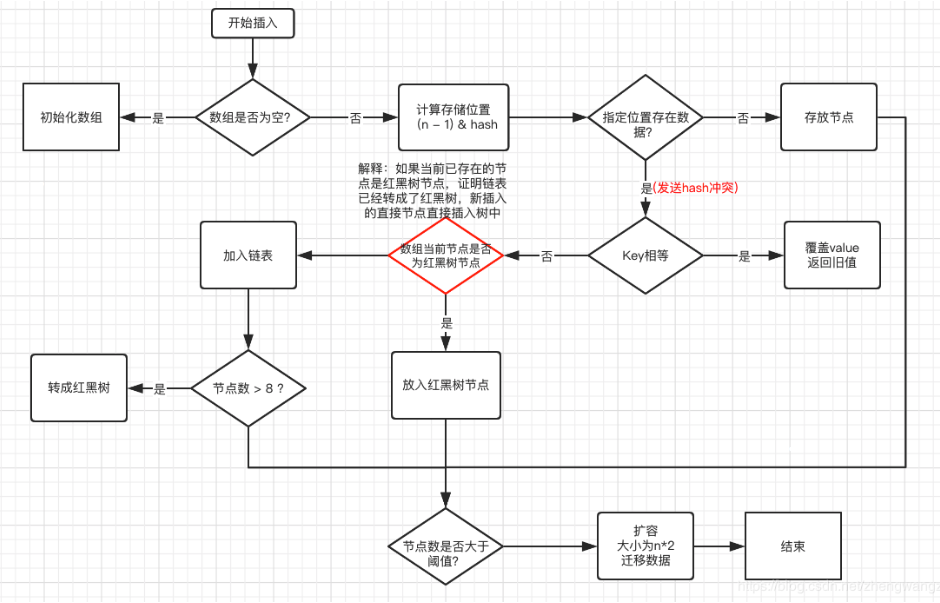public V put(K key, V value) {
//计算k的hash值
return putVal(hash(key), key, value, false, true);
}

// Node<K,V> p  确定的索引位置的键值对
// Node<K,V> e  新插入的键值对
// K k          索引位置的键值对的key
// int n        桶的长度
// int n, i      数组索引的位置
final V putVal(int hash, K key, V value, boolean onlyIfAbsent,
boolean evict) {
Node<K,V>[] tab; Node<K,V> p; int n, i;

// 1.校验table是否为空或者length等于0，如果是则调用resize方法进行初始化
if ((tab = table) == null || (n = tab.length) == 0)
n = (tab = resize()).length;

// 2.通过hash值计算索引位置，将该索引位置的头节点赋值给p，如果p为空则直接在该索引位置新增一个节点即可
if ((p = tab[i = (n - 1) & hash]) == null)
tab[i] = newNode(hash, key, value, null);
else {
// table表该索引位置不为空，则进行查找
Node<K,V> e; K k;
// 3.判断p节点的key和hash值是否跟传入的相等，如果相等, 则p节点即为要查找的目标节点，将p节点赋值给e节点
// k = p.key) == key  一般情况是用来比较 key为 null的情况
if (p.hash == hash && ((k = p.key) == key || (key != null && key.equals(k))))
e = p;
// 4.判断p节点是否为TreeNode, 如果是则调用红黑树的putTreeVal方法查找目标节点
else if (p instanceof TreeNode)
e = ((TreeNode<K,V>)p).putTreeVal(this, tab, hash, key, value);
else {
// 5.走到这代表p节点为普通链表节点，则调用普通的链表方法进行查找，使用binCount统计链表的节点数
for (int binCount = 0; ; ++binCount) {
// 6.如果p的next节点为空时，则代表找不到目标节点，则新增一个节点并插入链表尾部
if ((e = p.next) == null) {
p.next = newNode(hash, key, value, null);
// 7.当链表的长度大于等于树化阀值8，并且hash桶的长度大于等于MIN_TREEIFY_CAPACITY，如果超过则调用treeifyBin方法将链表节点转为红黑树节点，
// 减一是因为循环是从p节点的下一个节点开始的
if (binCount >= TREEIFY_THRESHOLD - 1)
treeifyBin(tab, hash);
break;
}
// 8.如果e节点存在hash值和key值都与传入的相同，则e节点即为目标节点，跳出循环
if (e.hash == hash &&
((k = e.key) == key || (key != null && key.equals(k))))
break;
p = e;  // 将p指向下一个节点，遍历连表
}
}
// 9.如果e节点不为空，则代表目标节点存在，使用传入的value覆盖该节点的value，并返回oldValue
if (e != null) {
V oldValue = e.value;
if (!onlyIfAbsent || oldValue == null)
e.value = value;
return oldValue;
}
}
++modCount;
// 10.如果插入节点后节点数超过阈值，则调用resize方法进行扩容
if (++size > threshold)
resize();
return null;
}


### 2.2.1、put–已经存返回旧的数据，添加新数据返回NULL

map.put("a","111");
System.out.println(map.put("a" , "aaa"));//111
System.out.println(map.put("b" , "bbb"));//NULL



### 2.2.2、红黑树相关

#### 2.2.2.1、putTreeVal，红黑树放入数据

1、如果该元素键的hash值小于当前节点的hash值的时候，就会作为当前节点的左节点，hash值大于当前节点hash值得时候作为当前节点的右节点。

2、如果hash值相同， 并且key也相等，则直接返回节点

3、如果hash值相同，但是key不相同，这时还是会先尝试看是否能够通过Comparable进行比较一下，要想看看是否能基于Comparable进行比较的话，首先要看该元素键是否实现了Comparable接口，此时就需要用到comparableClassFor方法来获取该元素键的Class。 否则定义一套规则来比较大小，确定是从左边查找树或者是右查找树

@Data
public class People  implements Comparable<People>{
private Integer id;
private String name;

@Override
public int compareTo(People p) {
return id - p.getId();
}
}


/**
* 红黑树的put操作，红黑树插入会同时维护原来的链表属性, 即原来的next属性
*/
final TreeNode<K,V> putTreeVal(HashMap<K,V> map, Node<K,V>[] tab,
int h, K k, V v) {
Class<?> kc = null;
boolean searched = false;

// 1.查找根节点, 索引位置的头节点并不一定为红黑树的根节点
TreeNode<K,V> root = (parent != null) ? root() : this;

// 2.将根节点赋值给p节点，开始进行查找
for (TreeNode<K,V> p = root;;) {
int dir, ph; K pk;

// 3.如果传入的hash值小于p节点的hash值，将dir赋值为-1，代表向p的左边查找树
if ((ph = p.hash) > h)
dir = -1;

// 4.如果传入的hash值大于p节点的hash值， 将dir赋值为1，代表向p的右边查找树
else if (ph < h)
dir = 1;

// 5.如果传入的hash值和key值等于p节点的hash值和key值, 则p节点即为目标节点, 返回p节点
else if ((pk = p.key) == k || (k != null && k.equals(pk)))
return p;

// 6.如果k所属的类没有实现Comparable接口 或者实现了该接口 k和p节点的key相等
else if ((kc == null && (kc = comparableClassFor(k)) == null) ||
(dir = compareComparables(kc, k, pk)) == 0) {

// 6.1 第一次符合条件, 从p节点的左节点和右节点分别调用find方法进行查找, 如果查找到目标节点则返回，找不到则定义一套规则来比较大小，决定左右子树
if (!searched) {
TreeNode<K,V> q, ch;
searched = true;
if (((ch = p.left) != null &&
(q = ch.find(h, k, kc)) != null) ||
((ch = p.right) != null &&
(q = ch.find(h, k, kc)) != null))
return q;
}

// 6.2 否则使用定义的一套规则来比较k和p节点的key的大小, 用来决定向左还是向右放入数据
dir = tieBreakOrder(k, pk); // dir<0则代表k<pk，则向p左边查找；反之亦然
}

TreeNode<K,V> xp = p;   // xp赋值为x的父节点,中间变量,用于下面给x的父节点赋值

// 7.dir<=0则向p左边查找,否则向p右边查找,如果为null,则代表该位置即为x的目标位置
if ((p = (dir <= 0) ? p.left : p.right) == null) {
// 走进来代表已经找到x的位置，只需将x放到该位置即可
Node<K,V> xpn = xp.next;    // xp的next节点

// 8.创建新的节点, 其中x的next节点为xpn, 即将x节点插入xp与xpn之间
TreeNode<K,V> x = map.newTreeNode(h, k, v, xpn);

// 9.调整x、xp、xpn之间的属性关系
if (dir <= 0)   // 如果时dir <= 0, 则代表x节点为xp的左节点
xp.left = x;
else        // 如果时dir> 0, 则代表x节点为xp的右节点
xp.right = x;
xp.next = x;    // 将xp的next节点设置为x
x.parent = x.prev = xp; // 将x的parent和prev节点设置为xp
// 如果xpn不为空,则将xpn的prev节点设置为x节点,与上文的x节点的next节点对应
if (xpn != null)
((TreeNode<K,V>)xpn).prev = x;

// 10.进行红黑树的插入平衡调整
moveRootToFront(tab, balanceInsertion(root, x));
return null;
}
}
}



#### 2.2.2.2、comparableClassFor 判断是否实现了Comparable


/**
* 如果对象x的类是C，如果C实现了Comparable<C>接口，那么返回C，否则返回null
*/
static Class<?> comparableClassFor(Object x) {
if (x instanceof Comparable) {
Class<?> c; Type[] ts, as; Type t; ParameterizedType p;
if ((c = x.getClass()) == String.class) // 如果x是个字符串对象
return c; // 返回String.class
/*
* 为什么如果x是个字符串就直接返回c了呢 ? 因为String  实现了 Comparable 接口，可参考如下String类的定义
* public final class String implements java.io.Serializable, Comparable<String>, CharSequence
*/

// 如果 c 不是字符串类，获取c直接实现的接口（如果是泛型接口则附带泛型信息）
if ((ts = c.getGenericInterfaces()) != null) {
for (int i = 0; i < ts.length; ++i) { // 遍历接口数组
// 如果当前接口t是个泛型接口
// 如果该泛型接口t的原始类型p 是 Comparable 接口
// 如果该Comparable接口p只定义了一个泛型参数
// 如果这一个泛型参数的类型就是c，那么返回c
if (((t = ts[i]) instanceof ParameterizedType) &&
((p = (ParameterizedType)t).getRawType() ==
Comparable.class) &&
(as = p.getActualTypeArguments()) != null &&
as.length == 1 && as == c) // type arg is c
return c;
}
// 上面for循环的目的就是为了看看x的class是否 implements  Comparable<x的class>
}
}
return null; // 如果c并没有实现 Comparable<c> 那么返回空



#### 2.2.2.3、compareComparables 比较是否相等

/**
* 如果x所属的类是kc，返回k.compareTo(x)的比较结果
* 如果x为空，或者其所属的类不是kc，返回0
*/
@SuppressWarnings({"rawtypes","unchecked"}) // for cast to Comparable
static int compareComparables(Class<?> kc, Object k, Object x) {
return (x == null || x.getClass() != kc ? 0 :
((Comparable)k).compareTo(x));
}



#### 2.2.2.4、tieBreakOrder 使用定义的一套规则来比较 k 和 p 节点的 key 的大小，用来决定向左还是向右查找

// 用于不可比较或者hashCode相同时进行比较的方法, 只是一个一致的插入规则，用来维护重定位的等价性。
static int tieBreakOrder(Object a, Object b) {
int d;
if (a == null || b == null ||
(d = a.getClass().getName().
compareTo(b.getClass().getName())) == 0)
d = (System.identityHashCode(a) <= System.identityHashCode(b) ?
-1 : 1);
return d;
}



#### 2.2.2.5、treeifyBin 链表转化为红黑树


/**
* 将链表节点转为红黑树节点
*/
final void treeifyBin(Node<K,V>[] tab, int hash) {
int n, index; Node<K,V> e;
// 1.如果table为空或者table的长度小于64, 调用resize方法进行扩容
if (tab == null || (n = tab.length) < MIN_TREEIFY_CAPACITY)
resize();
// 2.根据hash值计算索引值，将该索引位置的节点赋值给e，从e开始遍历该索引位置的链表
else if ((e = tab[index = (n - 1) & hash]) != null) {
TreeNode<K,V> hd = null, tl = null;
do {
// 3.将链表节点转红黑树节点
TreeNode<K,V> p = replacementTreeNode(e, null);
// 4.如果是第一次遍历，将头节点赋值给hd
if (tl == null)	// tl为空代表为第一次循环
hd = p;
else {
// 5.如果不是第一次遍历，则处理当前节点的prev属性和上一个节点的next属性
p.prev = tl;    // 当前节点的prev属性设为上一个节点
tl.next = p;    // 上一个节点的next属性设置为当前节点
}
// 6.将p节点赋值给tl，用于在下一次循环中作为上一个节点进行一些链表的关联操作（p.prev = tl 和 tl.next = p）
tl = p;
} while ((e = e.next) != null);
// 7.将table该索引位置赋值为新转的TreeNode的头节点，如果该节点不为空，则以以头节点(hd)为根节点, 构建红黑树
if ((tab[index] = hd) != null)
hd.treeify(tab);
}
}



#### 2.2.2.6.、treeify 构建红黑树

/**
* 构建红黑树
*/
final void treeify(Node<K,V>[] tab) {
TreeNode<K,V> root = null;
// 1.将调用此方法的节点赋值给x，以x作为起点，开始进行遍历
for (TreeNode<K,V> x = this, next; x != null; x = next) {
next = (TreeNode<K,V>)x.next;   // next赋值为x的下个节点
x.left = x.right = null;    // 将x的左右节点设置为空
// 2.如果还没有根节点, 则将x设置为根节点
if (root == null) {
x.parent = null;    // 根节点没有父节点
x.red = false;  // 根节点必须为黑色
root = x;   // 将x设置为根节点
}
else {
K k = x.key;	// k赋值为x的key
int h = x.hash;	// h赋值为x的hash值
Class<?> kc = null;
// 3.如果当前节点x不是根节点, 则从根节点开始查找属于该节点的位置
for (TreeNode<K,V> p = root;;) {
int dir, ph;
K pk = p.key;
// 4.如果x节点的hash值小于p节点的hash值，则将dir赋值为-1, 代表向p的左边查找
if ((ph = p.hash) > h)
dir = -1;
// 5.如果x节点的hash值大于p节点的hash值，则将dir赋值为1, 代表向p的右边查找
else if (ph < h)
dir = 1;
// 6.走到这代表x的hash值和p的hash值相等，则比较key值
else if ((kc == null && // 6.1 如果k没有实现Comparable接口 或者 x节点的key和p节点的key相等
(kc = comparableClassFor(k)) == null) ||
(dir = compareComparables(kc, k, pk)) == 0)
// 6.2 使用定义的一套规则来比较x节点和p节点的大小，用来决定向左还是向右查找
dir = tieBreakOrder(k, pk);

TreeNode<K,V> xp = p;   // xp赋值为x的父节点,中间变量用于下面给x的父节点赋值
// 7.dir<=0则向p左边查找,否则向p右边查找,如果为null,则代表该位置即为x的目标位置
if ((p = (dir <= 0) ? p.left : p.right) == null) {
// 8.x和xp节点的属性设置
x.parent = xp;  // x的父节点即为最后一次遍历的p节点
if (dir <= 0)   // 如果时dir <= 0, 则代表x节点为父节点的左节点
xp.left = x;
else    // 如果时dir > 0, 则代表x节点为父节点的右节点
xp.right = x;
// 9.进行红黑树的插入平衡(通过左旋、右旋和改变节点颜色来保证当前树符合红黑树的要求)
root = balanceInsertion(root, x);
break;
}
}
}
}
// 10.如果root节点不在table索引位置的头节点, 则将其调整为头节点
moveRootToFront(tab, root);
}



#### 2.2.2.7、moveRootToFront 红黑树平衡调整

/**
* 将root放到头节点的位置
* 如果当前索引位置的头节点不是root节点, 则将root的上一个节点和下一个节点进行关联,
* 将root放到头节点的位置, 原头节点放在root的next节点上
*/
static <K,V> void moveRootToFront(Node<K,V>[] tab, TreeNode<K,V> root) {
int n;
// 1.校验root是否为空、table是否为空、table的length是否大于0
if (root != null && tab != null && (n = tab.length) > 0) {
// 2.计算root节点的索引位置
int index = (n - 1) & root.hash;
TreeNode<K,V> first = (TreeNode<K,V>)tab[index];
// 3.如果该索引位置的头节点不是root节点，则该索引位置的头节点替换为root节点
if (root != first) {
Node<K,V> rn;
// 3.1 将该索引位置的头节点赋值为root节点
tab[index] = root;
TreeNode<K,V> rp = root.prev;   // root节点的上一个节点
// 3.2 和 3.3 两个操作是移除root节点的过程
// 3.2 如果root节点的next节点不为空，则将root节点的next节点的prev属性设置为root节点的prev节点
if ((rn = root.next) != null)
((TreeNode<K,V>)rn).prev = rp;
// 3.3 如果root节点的prev节点不为空，则将root节点的prev节点的next属性设置为root节点的next节点
if (rp != null)
rp.next = rn;
// 3.4 和 3.5 两个操作将first节点接到root节点后面
// 3.4 如果原头节点不为空, 则将原头节点的prev属性设置为root节点
if (first != null)
first.prev = root;
// 3.5 将root节点的next属性设置为原头节点
root.next = first;
// 3.6 root此时已经被放到该位置的头节点位置，因此将prev属性设为空
root.prev = null;
}
// 4.检查树是否正常
assert checkInvariants(root);
}
}



#### 2.2.2.8、checkInvariants 检查树是否正常

/**
* Recursive invariant check
*/
static <K,V> boolean checkInvariants(TreeNode<K,V> t) { // 一些基本的校验
TreeNode<K,V> tp = t.parent, tl = t.left, tr = t.right,
tb = t.prev, tn = (TreeNode<K,V>)t.next;
if (tb != null && tb.next != t)
return false;
if (tn != null && tn.prev != t)
return false;
if (tp != null && t != tp.left && t != tp.right)
return false;
if (tl != null && (tl.parent != t || tl.hash > t.hash))
return false;
if (tr != null && (tr.parent != t || tr.hash < t.hash))
return false;
if (t.red && tl != null && tl.red && tr != null && tr.red)  // 如果当前节点为红色, 则该节点的左右节点都不能为红色
return false;
if (tl != null && !checkInvariants(tl))
return false;
if (tr != null && !checkInvariants(tr))
return false;
return true;
}



## 2.3、get方法

1、计算hash值

2、判断hash桶是否为空，以及桶长度是否大于0，如果不成立直接返回并且是否该hash对应的数组索引位置是否有数据first，如果不成立，返回null，如果key相同，如果有数据并且key相同的话，直接返回，否则执行3

3、如果2不成立，并且该节点first有next值，则判断是否是红黑树节点，如果是红黑树节点，则去红黑树下查找，如果不是红黑树节点，则代表是链表，则去链表中查找。

4、如果2 3都不成立，则返回null

public V get(Object key) {
Node<K,V> e;
return (e = getNode(hash(key), key)) == null ? null : e.value;
}

final Node<K,V> getNode(int hash, Object key) {
Node<K,V>[] tab; Node<K,V> first, e; int n; K k;

// 1.对table进行校验：
//table不为空 && table长度大于0 && table索引位置(使用table.length - 1和hash值进行位与运算)的节点不为空,说明是有数据的
if ((tab = table) != null && (n = tab.length) > 0 && (first = tab[(n - 1) & hash]) != null) {

// 2.检查first节点的hash值和key是否和入参的一样，如果一样则first即为目标节点，直接返回first节点
if (first.hash == hash && ((k = first.key) == key || (key != null && key.equals(k))))
return first;

// 3.如果first不是目标节点，并且first的next节点不为空则继续遍历
if ((e = first.next) != null) {
if (first instanceof TreeNode)
// 4.如果是红黑树节点，则调用红黑树的查找目标节点方法getTreeNode
return ((TreeNode<K,V>)first).getTreeNode(hash, key);
do {

// 5.执行链表节点的查找，向下遍历链表, 直至找到节点的key和入参的key相等时,返回该节点
if (e.hash == hash &&
((k = e.key) == key || (key != null && key.equals(k))))
return e;
} while ((e = e.next) != null);
}
}
// 6.找不到符合的返回空
return null;
}


### 2.3.1、获取红黑树节点 getTreeNode

final TreeNode<K,V> getTreeNode(int h, Object k) {
// 1.首先找到红黑树的根节点；
// 2.使用根节点调用find方法
return ((parent != null) ? root() : this).find(h, k, null);
}


### 2.3.2、红黑树 find （不过多讲解，就是一个排序树）

/**
* 从调用此方法的节点开始查找, 通过hash值和key找到对应的节点
* 此方法是红黑树节点的查找, 红黑树是特殊的自平衡二叉查找树
* 平衡二叉查找树的特点：左节点<根节点<右节点
*/
final TreeNode<K,V> find(int h, Object k, Class<?> kc) {
// 1.将p节点赋值为调用此方法的节点，即为红黑树根节点
TreeNode<K,V> p = this;
// 2.从p节点开始向下遍历
do {
int ph, dir; K pk;
TreeNode<K,V> pl = p.left, pr = p.right, q;
// 3.如果传入的hash值小于p节点的hash值，则往p节点的左边遍历
if ((ph = p.hash) > h)
p = pl;
else if (ph < h) // 4.如果传入的hash值大于p节点的hash值，则往p节点的右边遍历
p = pr;
// 5.如果传入的hash值和key值等于p节点的hash值和key值,则p节点为目标节点,返回p节点
else if ((pk = p.key) == k || (k != null && k.equals(pk)))
return p;
else if (pl == null)    // 6.p节点的左节点为空则将向右遍历
p = pr;
else if (pr == null)    // 7.p节点的右节点为空则向左遍历
p = pl;

// 8.将p节点与k进行比较
else if ((kc != null ||
(kc = comparableClassFor(k)) != null) && // 8.1 kc不为空代表k实现了Comparable
(dir = compareComparables(kc, k, pk)) != 0)// 8.2 k<pk则dir<0, k>pk则dir>0
// 8.3 k<pk则向左遍历(p赋值为p的左节点), 否则向右遍历
p = (dir < 0) ? pl : pr;
// 9.代码走到此处, 代表key所属类没有实现Comparable, 直接指定向p的右边遍历（这样就不需要判断了自定规则了，没必要啊）
else if ((q = pr.find(h, k, kc)) != null)
return q;
// 10.代码走到此处代表“pr.find(h, k, kc)”为空, 因此直接向左遍历
else
p = pl;
} while (p != null);
return null;
}

8.将 p 节点与 k 进行比较。如果传入的 key（即代码中的参数 k）所属的类实现了 Comparable 接口（kc 不为空，comparableClassFor 方法见下面），

static Class<?> comparableClassFor(Object x) {
// 1.判断x是否实现了Comparable接口
if (x instanceof Comparable) {
Class<?> c; Type[] ts, as; Type t; ParameterizedType p;
// 2.校验x是否为String类型
if ((c = x.getClass()) == String.class) // bypass checks
return c;
if ((ts = c.getGenericInterfaces()) != null) {
// 3.遍历x实现的所有接口
for (int i = 0; i < ts.length; ++i) {
// 4.如果x实现了Comparable接口，则返回x的Class
if (((t = ts[i]) instanceof ParameterizedType) &&
((p = (ParameterizedType)t).getRawType() ==
Comparable.class) &&
(as = p.getActualTypeArguments()) != null &&
as.length == 1 && as == c) // type arg is c
return c;
}
}
}
return null;



## 2.4、resize方法（初始化hash桶也是用这个）

1、 判断旧的桶的最大容量是否为空，如果为空（初始化桶）则执行3，如果不为空，则表示桶里面现在是有数据的， 这个时候判断旧hash桶是否超过最大容量值：如果超过则将阈值设置为Integer.MAX_VALUE(2的30次幂)，并直接返回老表。这样就不会扩容了，一直往红黑树，链表，数组中放入数据，如果不超过最大容量，则表示可以扩容

2、 这时新的桶长度 = 旧的桶长度* 2 ，然后要求小于最大容量Integer.MAX_VALUE(2的30次幂) ，防止溢出，，并且旧桶长度 >= 初始的桶长度（16）, 则将新阈值设置为原来的两倍，总之目的就是得到新的桶的大小以及新阀值

3、如果旧的桶是空的，则表示要初始化桶，根据hashma初始化构造器的不同选择不同的if方式，总之目的就是得到新的桶的大小以及新阀值，因为是初始化不需要扩容，所以直接新桶就可以了

4、如果是2中的，需要扩容，扩容后节点重 hash 只可能分布在 “原索引位置” 与 “原索引 + oldCap。这样的话，如果是红黑树扩容的时候，可能遇到红黑树叶子变少的情况如果小于等于6，则红黑树变成链表

final Node<K,V>[] resize() {
Node<K,V>[] oldTab = table;
int oldCap = (oldTab == null) ? 0 : oldTab.length;
int oldThr = threshold;
//新的容量值，新的扩容阀界值
int newCap, newThr = 0;
// 如果旧hash桶不为空，说明是扩容
if (oldCap > 0) {

//如果此时oldCap>=MAXIMUM_CAPACITY(1 << 30)，表示已经到了最大容量，这时还要往map中放数据，则阈值设置为整数的最大值 Integer.MAX_VALUE，直接返回这个oldTab的内存地址。
if (oldCap >= MAXIMUM_CAPACITY) {
threshold = Integer.MAX_VALUE;
return oldTab;
}
// 将旧容量值<<1(相当于*2)赋值给 newCap
//这时新的桶长度 newCap <最大容量Integer.MAX_VALUE(2的30次幂) 如果等于或者大于的话，下面有一个代码会执行
//并且旧桶长度>=初始的桶长度DEFAULT_INITIAL_CAPACITY（16），olodCap小于DEFAULT_INITIAL_CAPACITY 的情况下面会执行
//操作：将原扩容阀界值<<1(相当于*2)赋值给 newThr，
else if ((newCap = oldCap << 1) < MAXIMUM_CAPACITY &&
oldCap >= DEFAULT_INITIAL_CAPACITY)
newThr = oldThr << 1; // double threshold
}

//进入此if证明创建map时用的带参构造：public HashMap(int initialCapacity)或 public HashMap(int initialCapacity, float loadFactor)
else if (oldThr > 0)
newCap = oldThr;

// 进入此if证明创建map时用的无参构造： public HashMap( )
else {
//3.老表的容量为0, 老表的阈值为0，这种情况是HashMap初始构造器没有传初始容量的new方法创建的空表，将阈值和容量设置为默认值 ，新的阀值  = 容量*负载因子
newCap = DEFAULT_INITIAL_CAPACITY;
}

//进入此if有两种可能
// 第一种：初始化，第一次put，说明是进入的有参数的,上面 “if (oldCap > 0) 初始化了newCap新桶容量，就等newThr新的阀值了
// 第二种：就是put元素的时候，要扩容，且旧容量小于oldCap 16
if (newThr == 0) {
float ft = (float)newCap * loadFactor;
newThr = (newCap < MAXIMUM_CAPACITY && ft < (float)MAXIMUM_CAPACITY ?
(int)ft : Integer.MAX_VALUE);
}

// 5.将当前阈值设置为刚计算出来的新的阈值，定义新表，容量为刚计算出来的新容量，将table设置为新定义的表。
threshold = newThr;
@SuppressWarnings({"rawtypes","unchecked"})
Node<K,V>[] newTab = (Node<K,V>[])new Node[newCap];
table = newTab;
// 6.如果老表不为空，则需遍历所有节点，将节点赋值给新表
if (oldTab != null) {
for (int j = 0; j < oldCap; ++j) {
Node<K,V> e;
if ((e = oldTab[j]) != null) {  // 将索引值为j的老表头节点赋值给e
oldTab[j] = null; // 将老表的节点设置为空, 以便垃圾收集器回收空间

// 7.如果e.next为空, 则代表老表的该位置只有1个节点，计算新表的索引位置, 直接将该节点放在该位置
if (e.next == null)
newTab[e.hash & (newCap - 1)] = e;

// 8.如果是红黑树节点，则进行红黑树的重hash分布(跟链表的hash分布基本相同)
else if (e instanceof TreeNode)
((TreeNode<K,V>)e).split(this, newTab, j, oldCap)
;
else { // preserve order
// 9.如果是普通的链表节点，则进行普通的重hash分布
Node<K,V> loHead = null, loTail = null; // 存储索引位置为:“原索引位置”的节点
Node<K,V> hiHead = null, hiTail = null; // 存储索引位置为:“原索引位置+oldCap”的节点
Node<K,V> next;
do {
next = e.next;
// 9.1 如果e的hash值与老表的容量进行与运算为0,则扩容后的索引位置跟老表的索引位置一样
// old 16=> 10000
if ((e.hash & oldCap) == 0) {
if (loTail == null) // 如果loTail为空, 代表该节点为第一个节点
else
loTail.next = e;    // 否则将节点添加在loTail后面
loTail = e; // 并将loTail赋值为新增的节点
}
// 9.2 如果e的hash值与老表的容量进行与运算为1,则扩容后的索引位置为:老表的索引位置＋oldCap
else {
if (hiTail == null) // 如果hiTail为空, 代表该节点为第一个节点
else
hiTail.next = e;    // 否则将节点添加在hiTail后面
hiTail = e; // 并将hiTail赋值为新增的节点
}
} while ((e = next) != null);

// 10.如果loTail不为空（说明老表的数据有分布到新表上“原索引位置”的节点），则将最后一个节点
// 的next设为空，并将新表上索引位置为“原索引位置”的节点设置为对应的头节点
if (loTail != null) {
loTail.next = null;
}

// 11.如果hiTail不为空（说明老表的数据有分布到新表上“原索引+oldCap位置”的节点），则将最后
// 一个节点的next设为空，并将新表上索引位置为“原索引+oldCap”的节点设置为对应的头节点
if (hiTail != null) {
hiTail.next = null;
}
}
}
}
}
// 12.返回新表
return newTab;



### 2.4.1、红黑树操作 split

/**
* 扩容后，红黑树的hash分布，只可能存在于两个位置：原索引位置、原索引位置+oldCap
*/
final void split(HashMap<K,V> map, Node<K,V>[] tab, int index, int bit) {
TreeNode<K,V> b = this;	// 拿到调用此方法的节点
TreeNode<K,V> loHead = null, loTail = null; // 存储索引位置为:“原索引位置”的节点
TreeNode<K,V> hiHead = null, hiTail = null; // 存储索引位置为:“原索引+oldCap”的节点
int lc = 0, hc = 0;
// 1.以调用此方法的节点开始，遍历整个红黑树节点
for (TreeNode<K,V> e = b, next; e != null; e = next) {	// 从b节点开始遍历
next = (TreeNode<K,V>)e.next;   // next赋值为e的下个节点
e.next = null;  // 同时将老表的节点设置为空，以便垃圾收集器回收
// 2.如果e的hash值与老表的容量进行与运算为0,则扩容后的索引位置跟老表的索引位置一样
if ((e.hash & bit) == 0) {
if ((e.prev = loTail) == null)  // 如果loTail为空, 代表该节点为第一个节点
else
loTail.next = e;    // 否则将节点添加在loTail后面
loTail = e; // 并将loTail赋值为新增的节点
++lc;   // 统计原索引位置的节点个数
}
// 3.如果e的hash值与老表的容量进行与运算为1,则扩容后的索引位置为:老表的索引位置＋oldCap
else {
if ((e.prev = hiTail) == null)  // 如果hiHead为空, 代表该节点为第一个节点
else
hiTail.next = e;    // 否则将节点添加在hiTail后面
hiTail = e; // 并将hiTail赋值为新增的节点
++hc;   // 统计索引位置为原索引+oldCap的节点个数
}
}
// 4.如果原索引位置的节点不为空
if (loHead != null) {   // 原索引位置的节点不为空
// 4.1 如果节点个数<=6个则将红黑树转为链表结构
if (lc <= UNTREEIFY_THRESHOLD)
else {
// 4.2 将原索引位置的节点设置为对应的头节点
// 已经被改变, 需要重新构建新的红黑树
}
}
// 5.如果索引位置为原索引+oldCap的节点不为空
if (hiHead != null) {   // 索引位置为原索引+oldCap的节点不为空
// 5.1 如果节点个数<=6个则将红黑树转为链表结构
if (hc <= UNTREEIFY_THRESHOLD)
else {
// 5.2 将索引位置为原索引+oldCap的节点设置为对应的头节点
// 已经被改变, 需要重新构建新的红黑树
}
}
}


### 2.4.2、untreeify红黑树转化为链表

/**
* 将红黑树节点转为链表节点, 当节点<=6个时会被触发
*/
final Node<K,V> untreeify(HashMap<K,V> map) {
Node<K,V> hd = null, tl = null; // hd指向头节点, tl指向尾节点
// 1.从调用该方法的节点, 即链表的头节点开始遍历, 将所有节点全转为链表节点
for (Node<K,V> q = this; q != null; q = q.next) {
// 2.调用replacementNode方法构建链表节点
Node<K,V> p = map.replacementNode(q, null);
// 3.如果tl为null, 则代表当前节点为第一个节点, 将hd赋值为该节点
if (tl == null)
hd = p;
// 4.否则, 将尾节点的next属性设置为当前节点p
else
tl.next = p;
tl = p; // 5.每次都将tl节点指向当前节点, 即尾节点
}
// 6.返回转换后的链表的头节点
return hd;
}


### 2.4.3、remove 方法

1、通过get那种方式先找到节点，如果数组节节点，则直接移除，如果是链表节点，则移除链表中的节点，如果是红黑树节点则移除，并判断是否满足红黑树转链表的两个条件，如果满足，则变成链表

/**
* 移除某个节点
*/
public V remove(Object key) {
Node<K,V> e;
return (e = removeNode(hash(key), key, null, false, true)) == null ?
null : e.value;
}

final Node<K,V> removeNode(int hash, Object key, Object value,
boolean matchValue, boolean movable) {
Node<K,V>[] tab; Node<K,V> p; int n, index;
// 1.如果table不为空并且根据hash值计算出来的索引位置不为空, 将该位置的节点赋值给p
if ((tab = table) != null && (n = tab.length) > 0 &&
(p = tab[index = (n - 1) & hash]) != null) {
Node<K,V> node = null, e; K k; V v;
// 2.如果p的hash值和key都与入参的相同, 则p即为目标节点, 赋值给node
if (p.hash == hash &&
((k = p.key) == key || (key != null && key.equals(k))))
node = p;
else if ((e = p.next) != null) {
// 3.否则将p.next赋值给e，向下遍历节点
// 3.1 如果p是TreeNode则调用红黑树的方法查找节点
if (p instanceof TreeNode)
node = ((TreeNode<K,V>)p).getTreeNode(hash, key);
else {
// 3.2 否则，进行普通链表节点的查找
do {
// 当节点的hash值和key与传入的相同,则该节点即为目标节点
if (e.hash == hash &&
((k = e.key) == key ||
(key != null && key.equals(k)))) {
node = e;	// 赋值给node, 并跳出循环
break;
}
p = e;  // p节点赋值为本次结束的e，在下一次循环中，e为p的next节点
} while ((e = e.next) != null); // e指向下一个节点
}
}
// 4.如果node不为空(即根据传入key和hash值查找到目标节点)，则进行移除操作
if (node != null && (!matchValue || (v = node.value) == value ||
(value != null && value.equals(v)))) {
// 4.1 如果是TreeNode则调用红黑树的移除方法
if (node instanceof TreeNode)
((TreeNode<K,V>)node).removeTreeNode(this, tab, movable);
// 4.2 如果node是该索引位置的头节点则直接将该索引位置的值赋值为node的next节点，
// “node == p”只会出现在node是头节点的时候，如果node不是头节点，则node为p的next节点
else if (node == p)
tab[index] = node.next;
// 4.3 否则将node的上一个节点的next属性设置为node的next节点,
// 即将node节点移除, 将node的上下节点进行关联(链表的移除)
else
p.next = node.next;
++modCount;
--size;
// 5.返回被移除的节点
return node;
}
}
return null;
}



### 2.4.4、红黑树 removeTreeNode

**
* 红黑树的节点移除
*/
final void removeTreeNode(HashMap<K,V> map, Node<K,V>[] tab,
boolean movable) {
// --- 链表的处理start ---
int n;
// 1.table为空或者length为0直接返回
if (tab == null || (n = tab.length) == 0)
return;
// 2.根据hash计算出索引的位置
int index = (n - 1) & hash;
// 3.将索引位置的头节点赋值给first和root
TreeNode<K,V> first = (TreeNode<K,V>)tab[index], root = first, rl;
// 4.该方法被将要被移除的node(TreeNode)调用, 因此此方法的this为要被移除node节点,
// 将node的next节点赋值给succ节点，prev节点赋值给pred节点
TreeNode<K,V> succ = (TreeNode<K,V>)next, pred = prev;
// 5.如果pred节点为空，则代表要被移除的node节点为头节点，
// 则将table索引位置的值和first节点的值赋值为succ节点(node的next节点)即可
if (pred == null)
tab[index] = first = succ;
else
// 6.否则将pred节点的next属性设置为succ节点(node的next节点)
pred.next = succ;
// 7.如果succ节点不为空，则将succ的prev节点设置为pred, 与前面对应
if (succ != null)
succ.prev = pred;
// 8.如果进行到此first节点为空，则代表该索引位置已经没有节点则直接返回
if (first == null)
return;
// 9.如果root的父节点不为空, 则将root赋值为根节点
if (root.parent != null)
root = root.root();
// 10.通过root节点来判断此红黑树是否太小, 如果是则调用untreeify方法转为链表节点并返回
// (转链表后就无需再进行下面的红黑树处理)
if (root == null || root.right == null ||
(rl = root.left) == null || rl.left == null) {
tab[index] = first.untreeify(map);  // too small
return;
}
// --- 链表的处理end ---

// --- 以下代码为红黑树的处理 ---
// 11.将p赋值为要被移除的node节点，pl赋值为p的左节点，pr赋值为p 的右节点
TreeNode<K,V> p = this, pl = left, pr = right, replacement;
// 12.如果p的左节点和右节点都不为空时
if (pl != null && pr != null) {
// 12.1 将s节点赋值为p的右节点
TreeNode<K,V> s = pr, sl;
// 12.2 向左一直查找，跳出循环时,s为没有左节点的节点
while ((sl = s.left) != null)
s = sl;
// 12.3 交换p节点和s节点的颜色
boolean c = s.red; s.red = p.red; p.red = c;
TreeNode<K,V> sr = s.right; // s的右节点
TreeNode<K,V> pp = p.parent;    // p的父节点
// --- 第一次调整和第二次调整：将p节点和s节点进行了位置调换 ---
// 12.4 第一次调整
// 如果p节点的右节点即为s节点，则将p的父节点赋值为s，将s的右节点赋值为p
if (s == pr) {
p.parent = s;
s.right = p;
}
else {
// 将sp赋值为s的父节点
TreeNode<K,V> sp = s.parent;
// 将p的父节点赋值为sp
if ((p.parent = sp) != null) {
// 如果s节点为sp的左节点，则将sp的左节点赋值为p节点
if (s == sp.left)
sp.left = p;
// 否则s节点为sp的右节点，则将sp的右节点赋值为p节点
else
sp.right = p;
}
// s的右节点赋值为p节点的右节点
if ((s.right = pr) != null)
// 如果pr不为空，则将pr的父节点赋值为s
pr.parent = s;
}
// 12.5 第二次调整
// 将p的左节点赋值为空，pl已经保存了该节点
p.left = null;
// 将p节点的右节点赋值为sr，如果sr不为空，则将sr的父节点赋值为p节点
if ((p.right = sr) != null)
sr.parent = p;
// 将s节点的左节点赋值为pl，如果pl不为空，则将pl的父节点赋值为s节点
if ((s.left = pl) != null)
pl.parent = s;
// 将s的父节点赋值为p的父节点pp
// 如果pp为空，则p节点为root节点, 交换后s成为新的root节点
if ((s.parent = pp) == null)
root = s;
// 如果p不为root节点, 并且p是pp的左节点，则将pp的左节点赋值为s节点
else if (p == pp.left)
pp.left = s;
// 如果p不为root节点, 并且p是pp的右节点，则将pp的右节点赋值为s节点
else
pp.right = s;
// 12.6 寻找replacement节点，用来替换掉p节点
// 12.6.1 如果sr不为空，则replacement节点为sr，因为s没有左节点，所以使用s的右节点来替换p的位置
if (sr != null)
replacement = sr;
// 12.6.1 如果sr为空，则s为叶子节点，replacement为p本身，只需要将p节点直接去除即可
else
replacement = p;
}
// 13.承接12点的判断，如果p的左节点不为空，右节点为空，replacement节点为p的左节点
else if (pl != null)
replacement = pl;
// 14.如果p的右节点不为空,左节点为空，replacement节点为p的右节点
else if (pr != null)
replacement = pr;
// 15.如果p的左右节点都为空, 即p为叶子节点, replacement节点为p节点本身
else
replacement = p;
// 16.第三次调整：使用replacement节点替换掉p节点的位置，将p节点移除
if (replacement != p) { // 如果p节点不是叶子节点
// 16.1 将p节点的父节点赋值给replacement节点的父节点, 同时赋值给pp节点
TreeNode<K,V> pp = replacement.parent = p.parent;
// 16.2 如果p没有父节点, 即p为root节点，则将root节点赋值为replacement节点即可
if (pp == null)
root = replacement;
// 16.3 如果p不是root节点, 并且p为pp的左节点，则将pp的左节点赋值为替换节点replacement
else if (p == pp.left)
pp.left = replacement;
// 16.4 如果p不是root节点, 并且p为pp的右节点，则将pp的右节点赋值为替换节点replacement
else
pp.right = replacement;
// 16.5 p节点的位置已经被完整的替换为replacement, 将p节点清空, 以便垃圾收集器回收
p.left = p.right = p.parent = null;
}
// 17.如果p节点不为红色则进行红黑树删除平衡调整
// (如果删除的节点是红色则不会破坏红黑树的平衡无需调整)
TreeNode<K,V> r = p.red ? root : balanceDeletion(root, replacement);

// 18.如果p节点为叶子节点, 则简单的将p节点去除即可
if (replacement == p) {
TreeNode<K,V> pp = p.parent;
// 18.1 将p的parent属性设置为空
p.parent = null;
if (pp != null) {
// 18.2 如果p节点为父节点的左节点，则将父节点的左节点赋值为空
if (p == pp.left)
pp.left = null;
// 18.3 如果p节点为父节点的右节点， 则将父节点的右节点赋值为空
else if (p == pp.right)
pp.right = null;
}
}
if (movable)
// 19.将root节点移到索引位置的头节点
moveRootToFront(tab, r);
}



## 2.5、最终总结

### 2.5.1、hashmap时间复杂度为O(1)

Ο(1)＜Ο(log2n)＜Ο(n)＜Ο(nlog2n)＜Ο(n2)<Ο(n3)＜…＜Ο(n^2)＜Ο(n!)

#### 2.5.1.1、取值

1.判断key，根据key算出索引。

2.根据索引获得索引位置所对应的键值对链表。

3.遍历键值对链表/红黑树，根据key找到对应的Entry键值对。

4.拿到value。

### 2.5.1、扩容hash分布原理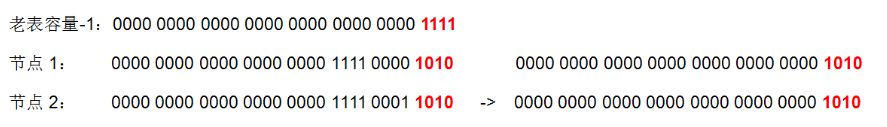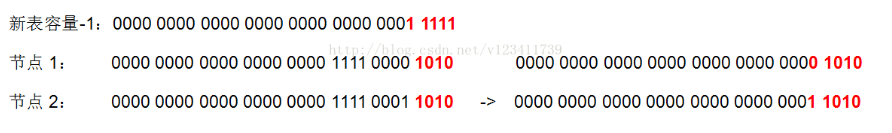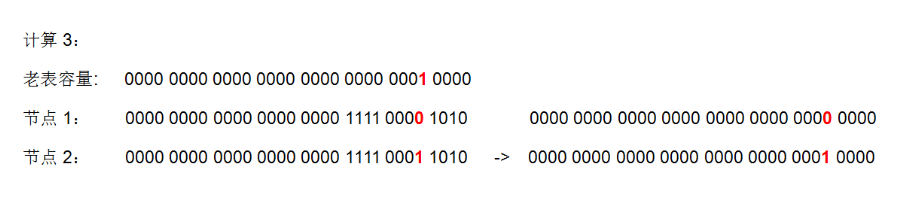bit =  oldCap ；
if ((e.hash & bit) == 0) {
if ((e.prev = loTail) == null)  // 如果loTail为空, 代表该节点为第一个节点
else
loTail.next = e;    // 否则将节点添加在loTail后面
loTail = e; // 并将loTail赋值为新增的节点
++lc;   // 统计原索引位置的节点个数
}


### 2.5.3、HashMap为什么右移16和使用 & 与运算代替模运算

hash值是为了获取数组下标的，很明显就知道该hash值是为了均匀散列表的下标，仔细看看，就知道下面使用了 hashcode 右移16位再和自己异或得到的hash值

// 代码1
static final int hash(Object key) { // 计算key的hash值
int h;
// 1.先拿到key的hashCode值; 2.将hashCode的高16位参与运算
return (key == null) ? 0 : (h = key.hashCode()) ^ (h >>> 16);
}

// 代码2
int n = tab.length;

// 将(tab.length - 1) 与 hash值进行&运算
int index = (n - 1) & hash;



1、拿到 key 的 hashCode 值

2、将 hashCode 的高位参与运算，重新计算 hash 值

3、将计算出来的 hash 值与 (table.length - 1) 进行 & 运算

static final int hash(Object key) {
int h;
return (key == null) ? 0 : (h = key.hashCode()) ^ (h >>> 16);
}


#### 2.5.3.1、为什么要右移16位

15 的二进制数 0000 0000 0000 0000 0000 0000 0000 1111 ，发现结果都是0。这样的话数组小标就都是0了，这样的结果应该不是我们想看到的，只使用了hashcode的低4位，没有充分利用hashcde，如果数据足够多，这种重复的数据还是挺多的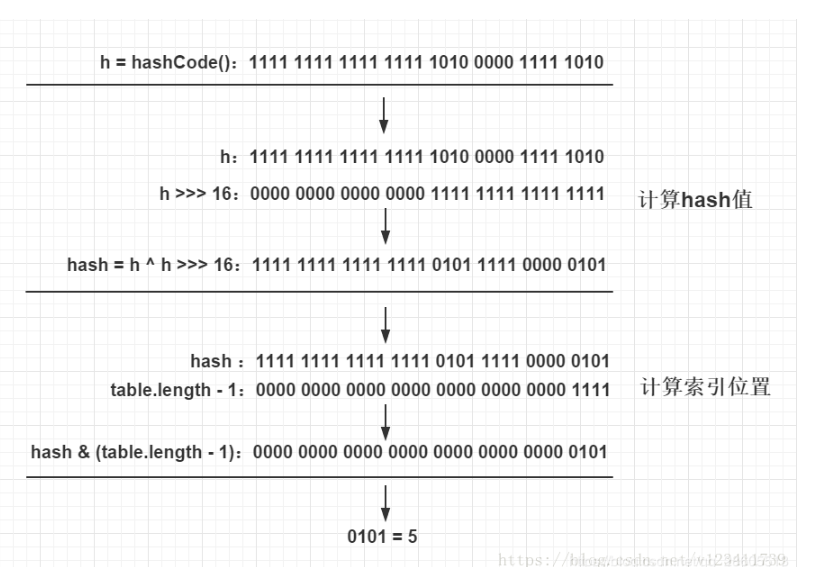#### 2.5.3.2、HashMaps索引为什么使用 & 与运算代替模运算

tab[ (n - 1) & hash   ]；


1、除法和求余数（模运算）是最慢的动作：其中 n 是数组的长度。其实该算法的结果和模运算的结果是相同的。但是，对于现代的处理器来说， （机器中都是二进制存储的，计算的起始也是二进制计算的，如果是除法或者其他模运算，还要转化成2进制再运算。增加劳动力，何必呢）

2、充分利用hash值：当 n 为 2 的幂次方的时候，n-1的二进制会得到 一堆1111…… 的数字，这个数字正好可以掩码 （都是一堆 1111……），并且得到的结果取决于 hash 值。因为 hash 位值是1，那么最终的结果也是1 ，hash位 值是0，最终的结果也是0。

### 2.5.4、HashMap 的容量为什么建议是 2的幂次方？

hash 算法的目的是为了让hash值均匀的分布在桶中（数组），那么，如何做到呢？试想一下，如果不使用 2 的幂次方作为数组的长度会怎么样？

1001 & 101010100101001001000 结果：1000 = 8

1001 & 101000101101001001001 结果：1001 = 9

1001 & 101010101101101001010 结果： 1000 = 8

1001 & 101100100111001101100 结果： 1000 = 8

### 2.5.5、我们自定义 HashMap 容量最好是多少

1、比如链表数很多，肯定是数组初始化长度不对，这样的话会造成拥挤

2、如果某个map很大，注意，肯定是事先没有定义好初始化长度

### 2.5.6、使用指南

1、允许NULL值，NULL键

2、扩容的阀值threshold，当键值对的数量超过这个阀值会产生扩容， Hash数组 每次会扩容长度为以前的2倍

3、 不要轻易改变负载因子，负载因子过高（不会轻易扩容）会导致链表过长，查找键值对时间复杂度就会增高，负载因子过低（一下子就会扩容了）会导致hash桶的 数量过多，空间复杂度会增高

4、HashMap是多线程不安全

5、尽量设置HashMap的初始容量，尤其在数据量大的时候，防止多次resize

### 2.5.7、Jdk1.8 和 Jdk1.7中 hashmap区别

JDK1.7进行多线程put操作，之后遍历，直接死循环，CPU飙到100%JDK1.8中进行多线程操作会出现节点和value值丢失

JDK1.8中HashMap使用高低位来平移元素，这样保证效率的同时避免了多线程情况下扩容造成死循环的问题。

#### 2.5.7.1、jdk1.7链表是如何死循环的

##### JDK1.7、正常的ReHash的过程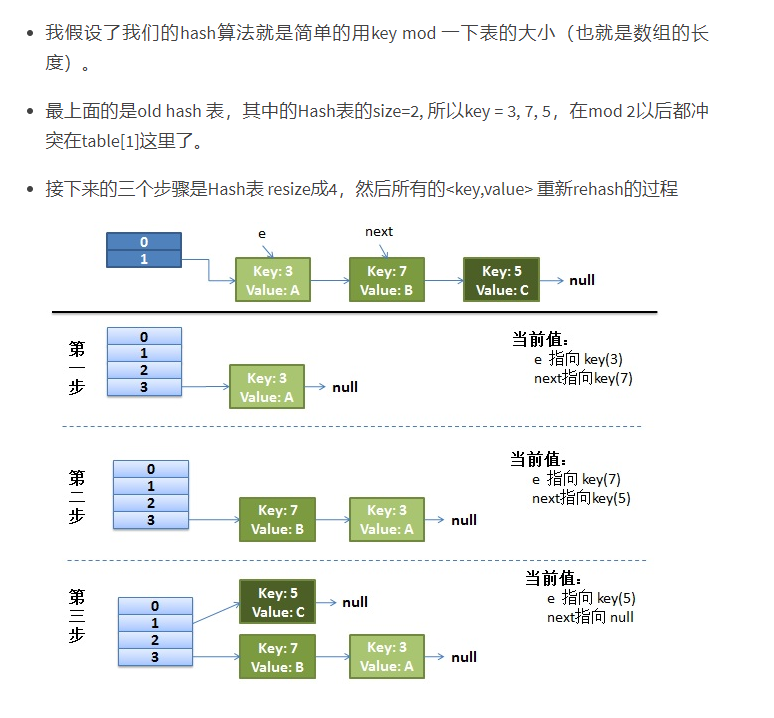##### JDK1.7、并发的ReHash的过程

1）假设我们有两个线程

do {
Entry<K,V> next = e.next; // <--假设线程一执行到这里就被调度挂起了，这行已经执行了哦
int i = indexFor(e.hash, newCapacity);
e.next = newTable[i];//将原来数组当前位置放到新节点后面
newTable[i] = e;//将新的节点设置为当前数组位置上的值
e = next;
} while (e != null);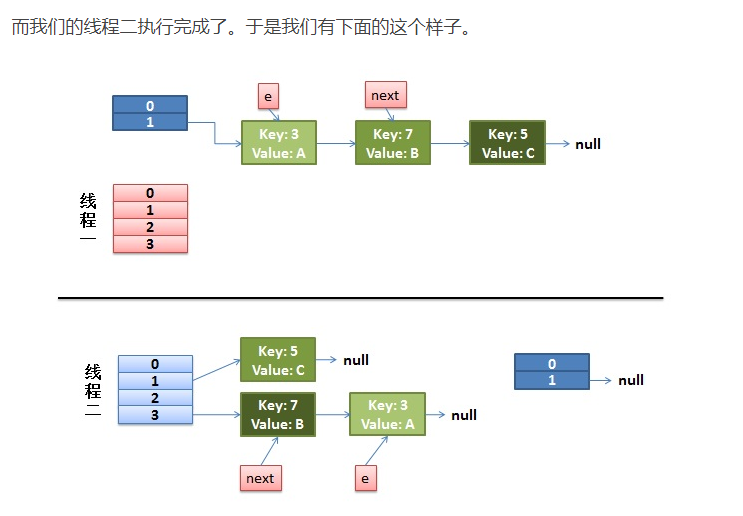2）线程一被调度回来执行。

do {
Entry<K,V> next = e.next; // //此行代码已经执行了  ，线程1已经获取到e的值为key3，e.next=key7
int i = indexFor(e.hash, newCapacity);
e.next = newTable[i];//现在数组当前位置上的newTable[i]值为key7，->将key3的next设置为key7
newTable[i] = e; //再将key3设置为当前数组位置的值。注意了，这个时候，key7的next还是key3，这样就导致了闭环出现了
e = next;
} while (e != null);
`